Friday, January 22, 2021
Home > download jstse papers > JSTSE CHAPTER WISE QUESTIONS OF MATHEMATICS

# JSTSE CHAPTER WISE QUESTIONS OF MATHEMATICS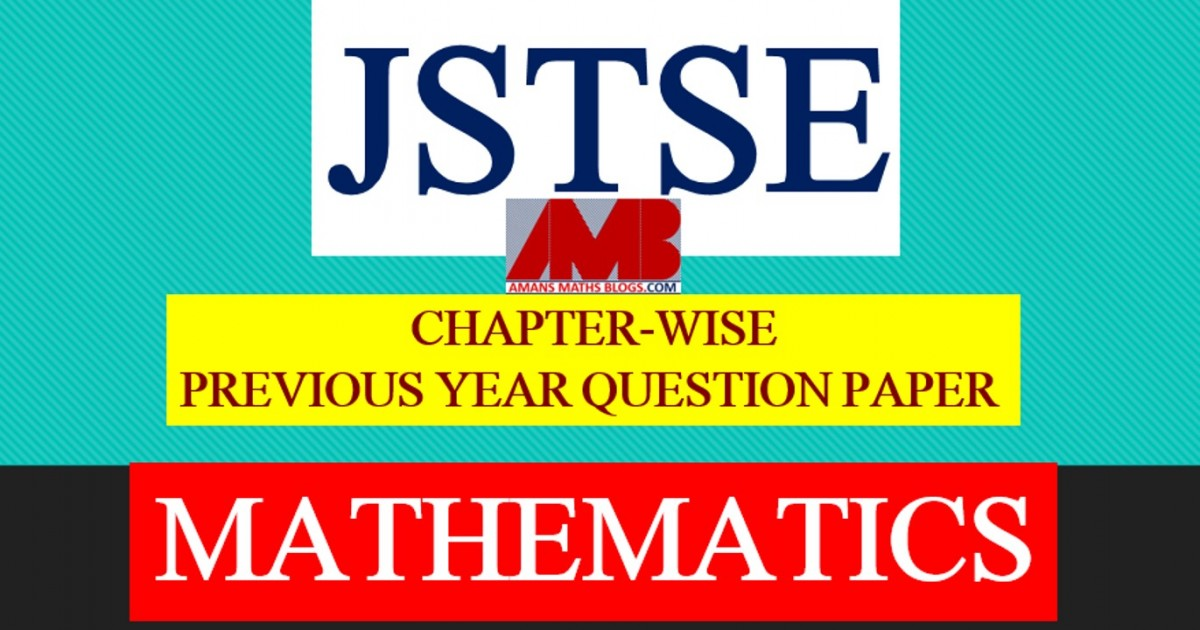# JSTSE Chapter Wise Questions of Mathematics

JSTSE (Junior Science Talent Search Examination) is an exam conducted by Science Branch of DTE of Education, Delhi. JSTSE exam is scheduled every year in the month of January for the students of recognized schools of Delhi. In this post, you will get JSTSE Chapter wise questions of Mathematics.

JSTSE
Previous Year Question Papers
2007-082008-092009-102010-112011-12
2012-132013-142014-152015-162016-17
2018-19PhysicsChemistryMathsBiology

## JSTSE Question Papers : Mathematics Chapter 1 : Algebraic Identities

Some of questions of JSTSE Maths Question Paper Chapter 1: Algebraic Identities as below

Read More : 129 Maths Short Tricks

JSTSE Chapter wise Questions of Mathematics Ques No 1:

Factors of (x2/2) – (y2/3) are

Options:

A. (x/Root(2) + y/Root(3))(x/Root(2) + y/Root(3))

B. (x/2 + y/3)(x/2 – y/3)

C. (x/Root(2) – y/Root(3))(x/Root(2) – y/Root(3))

D. (x/Root(2) + y/Root(3))(x/Root(2) – y/Root(3))

JSTSE Maths Question Paper Ques No 2:

If Root(x / (x + 3)) = 2-1, then the value of x is

Options:

A. 1/6

B. 6

C. 1

D. 3

JSTSE Chapter wise Questions of Mathematics Ques No 3:

If x + 1/x = 2, then x – 1/x is

Options:

A. -1

B. 0

C. 1

D. 4

JSTSE Maths Question Paper Ques No 4:

If x = 1/ (2 – Root(3)), then the value of x2 – 4x + 11 is

Options:

A. 10.268

B. 10

C. 0.732

D. 0.268

JSTSE Chapter wise Questions of Mathematics Ques No 5:

If x = 1 – Root(2), then the value of (x – 1/x)3 is

Options:

A. 8

B. 8 + Root(2)

C. 8 – Root(2)

D. 3 – Root(2)

For all questions of JSTSE Maths Question Paper Chapter 1: Algebraic IdentitiesClick Here

## JSTSE Question Papers : Mathematics Chapter 2 : Area

Some of questions of JSTSE Maths Question Paper Chapter 2: Area as below

JSTSE Chapter wise Questions of Mathematics Ques No 1:

AD is the median of ∆ABC and DE is median of ∆ABD. If ar(∆ABC) = 40 cm2, then the value of ar(∆BDE) is

Options:

A. 13.33 cm2

B. 20 cm2

C. 10 cm2

D. None of these

JSTSE Maths Question Paper Ques No 2:

ABCD is a parallelogram X and Y are mid points of BC and CD respectively, then the ar(∆CYX) will be

Options:

A. (1/4) ar(ABCD)

B. (3/4) ar(ABCD)

C. (1/8) ar(ABCD)

D. (3/8) ar(ABCD)

JSTSE Chapter wise Questions of Mathematics Ques No 3:

A triangular region is formed in the first quadrant by the line y = 2, x = 6 and y = x. The area of this triangular region is

Options:

A. 4 sq units

B. 6 sq units

C. 8 sq units

D. 18 sq units

JSTSE Maths Question Paper Ques No 4:

In the given figure, AB = BC = 7 cm and DE = 2 cm, then the shaded area of the figure is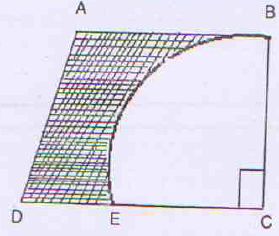Options:

A. 22.5 cm2

B. 38.5 cm2

C. 17.5 cm2

D. None of above

JSTSE Chapter wise Questions of Mathematics Ques No 5:

AD is the median of ∆ABC and DE is the median of ∆ABD. If area of ∆ABC is 80 cm2, then the area of ACDE is

Options:

A. 66.3 cm2

B. 60 cm2

C. 40 cm2

D. None of above

For all questions of JSTSE Maths Question Paper Chapter 2: AreaClick Here

## JSTSE Question Papers : Mathematics Chapter 3 : Arithmetic Progression

Some of questions of JSTSE Maths Question Paper Chapter 3: Arithmetic Progression as below

JSTSE Chapter wise Questions of Mathematics Ques No 1:

The first and last term of an AP are a and l respectively. If S is the sum of all terms of AP and common difference is given by (l2 – a2)/[k – (l + a)], then the value of k will be

Options:

A. S

B. 2S

C. 3S

D. None of above

JSTSE Maths Question Paper Ques No 2:

Autorikshaw fare in a city is Rs. 20 for first two kilometer and Rs. 6 per kilometer for subsequent distances covered. Taking the distance covered as x km and total fare as Rs. y, the linear equation in which expresses the above statement is

Options:

A. y = 6x + 8

B. y = 6x – 8

C. y = 6x + 20

D. y = 6x + 28

JSTSE Chapter wise Questions of Mathematics Ques No 3:

If x = (-23) + 22 + (-23) + 22 + … to 40 terms and y = 11 + (-10) + 11 + (-10) + … to 20 terms, then the value of (y – x)

Options:

A. 30

B. 40

C. 20

D. 10

JSTSE Maths Question Paper Ques No 4:

The sum of five consecutive odd natural numbers is 65. Then, the sum of extreme numbers is

Options:

A. 24

B. 26

C. 28

D. 30

For all questions of JSTSE Maths Question Paper Chapter 3: Arithmetic ProgressionClick Here

## JSTSE Question Papers : Mathematics Chapter 4 : Circle

Some of questions of JSTSE Maths Question Paper Chapter 4: Circle as below

JSTSE Chapter wise Questions of Mathematics Ques No 1:

The area of a circular ring enclosed between two concentric circles is 286 cm2. If the difference between their radii is 7 cm, then the radii of these circles are

Options:

A. 12 cm and 5 cm

B. 9 cm and 2 cm

C. 11 cm and 4 cm

D. 10 cm and 3 cm

JSTSE Maths Question Paper Ques No 2:

In figure, if BC passes through the center of the circle and AB = AC = a units, then the area of the shaded region isOptions:

A. (a2/2)(3 – π)

B. a2(π/2 – 1)

C. 2a2(π – 1)

D. (a2/2)(π/2 – 1)

JSTSE Chapter wise Questions of Mathematics Ques No 3:

In the figure, there are three semi-circles. The radii of both smaller semi-circles is equal. If the radius of the larger semi-circle is 22 cm, then the area of shaded region is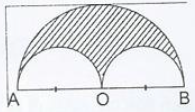Options:

A. 363π/4 cm2

B. 121π cm2

C. 236.5π cm2

D. 363π cm2

JSTSE Maths Question Paper Ques No 4:

In the given figure, ∠AQB is equal to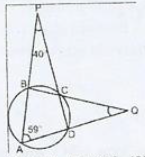Options:

A. 18 degree

B. 20 degree

C. 22 degree

D. 24 degree

JSTSE Chapter wise Questions of Mathematics Ques No 5:

In figure, O is the centre of the circle, then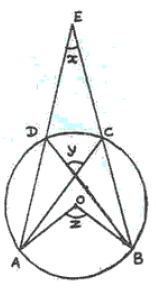Options:

A. x + y + z = 180 degree

B. z = 2x + y

C. y = z + x

D. z = x + y

For all questions of JSTSE Maths Question Paper Chapter 4: CircleClick Here

## JSTSE Question Papers : Mathematics Chapter 5 : Coordinate Geometry

Some of questions of JSTSE Maths Question Paper Chapter 5: Coordinate Geometry as below

JSTSE Chapter wise Questions of Mathematics Ques No 1:

Which of the following points does not lie on a circle whose center is at O(0, 0) and radius is 5Root(2):

Options:

A. (7, -1)

B. (-5, 5)

C. (5, -7)

D. (-1, 7)

JSTSE Maths Question Paper Ques No 2:

The graph of a equation is a line parallel to x-axis at a distance 5/2 below it. That equation is

Options:

A. 2x + 5 = 0

B. 2y + 5 = 0

C. y = x – 5/2

D. y = x + 5/2

JSTSE Chapter wise Questions of Mathematics Ques No 3:

The distance between points A(2, 0) and B(6, 3) is

Options:

A. 7 units

B. 6 units

C. 5 units

D. 4 units

JSTSE Maths Question Paper Ques No 4:

In a square PQRS, if P(1, 0), Q(4, 0) and S(1, 3) then the coordinates of point R will be

Options:

A. (4, 1)

B. (4, 4)

C. (3, 4)

D. (4, 3)

JSTSE Chapter wise Questions of Mathematics Ques No 5:

The equations 1/x + 1/y = 15 and 1/x – 1/y = 5 are such that ax = 1 and by = 1. The values of a and b respectively are:

Options:

A. 10, 5

B. 10, -5

C. -5, 10

D. 5, 10

For all questions of JSTSE Maths Question Paper Chapter 5: Coordinate GeometryClick Here

## JSTSE Question Papers : Mathematics Chapter 6 : Direct & Inverse Proportion

Some of questions of JSTSE Maths Question Paper Chapter 6: Direct & Inverse Proportion as below

JSTSE Chapter wise Questions of Mathematics Ques No 1:

Two numbers are in the ratio 3 : 1 and their sum is 28. In order to get the ratio of 1 : 3, then the second number have to increased by

Options:

A. 28

B. 35

C. 56

D. 84

JSTSE Maths Question Paper Ques No 2:

The percentage of marks obtained by a students in the monthly unit test are given below. The probability that the students gets at least 60% marks is

Options:

A. 0

B. 2/3

C. 3/5

D. 4/5

JSTSE Chapter wise Questions of Mathematics Ques No 3:

A tap can fill an empty tank in 12 hours and a leakage can empty the whole tank in  20 hours. If the tap and the leakage are working simultaneously the whole tank to fill will take

Options:

A. 25 hrs

B. 40 hrs

C. 30 hrs

D. 35 hrs

JSTSE Maths Question Paper Ques No 4:

By travelling at 4/5th of his usual speed a person is late by 20 minutes. Initial time to cover the distance was

Options:

A. 80 minutes

B. 65 minutes

C. 45 minutes

D. 50 minutes

JSTSE Chapter wise Questions of Mathematics Ques No 5:

In a mixture of 75 litres, the ratio of milk of water is 2 : 1. The amount of water to be further added to the mixture so as to wake the ratio of milk to water 1 : 2  will be

Options:

A. 60 lts.

B. 45 lts.

C. 75 lts.

D. 30 lts.

For all questions of JSTSE Maths Question Paper Chapter 6: Direct & Inverse ProportionClick Here

## JSTSE Question Papers : Mathematics Chapter 7 : Exponents & Powers

Some of questions of JSTSE Maths Question Paper Chapter 7: Exponents & Powers as below

JSTSE Chapter wise Questions of Mathematics Ques No 1:

Simplification (16 x 2n+1 – 4 x 2n) / (16 x 2n+2 – 2 x 2n+1) is

Options:

A. 12n / (14n + 1)

B. 12 x 2n / (14 x 2n+1)

C. 2/7

D. 1/2

JSTSE Maths Question Paper Ques No 2:

The value of (1-1 + 2-1 – 3-1) / 2 is

Options:

A. 11/12

B. 12/11

C. 1/6

D. 2/5

JSTSE Chapter wise Questions of Mathematics Ques No 3:

If 1/x = 2, 1/y = 3, 1/z = 4, then the value of (x-2 + y-2 + z-2)-1 will be

Options:

A. (1/9)-1

B. (1/19)-1

C. (1/29)-1

D. 1/29

JSTSE Maths Question Paper Ques No 4:

If x, y, z, are positive real numbers, then the value of √(x-1y) √(y-1z) √(z-1x) will be

Options:

A. xyz

B. x-1y-1z-1

C. 1

D. 0

JSTSE Chapter wise Questions of Mathematics Ques No 5:

If 29x = 1, then x will be

Options:

A. Non Negative Integer

B. 0

C. Not Defined

D. None of above

For all questions of JSTSE Maths Question Paper Chapter 7: Exponents & powersClick Here

## JSTSE Question Papers : Mathematics Chapter 8 : Linear Equations

Some of questions of JSTSE Maths Question Paper Chapter 8: Linear Equations as below

JSTSE Chapter wise Questions of Mathematics Ques No 1:

How much pure alcohol should be added to 400 ml of a 15% solution to make its strength 32% ?

Options:

A. 80 ml

B. 90 ml

C. 95 ml

D. 100 ml

JSTSE Maths Question Paper Ques No 2:

A boy is running at a speed of p km/h to cover a distance of 1 km. But due to slippery ground, his speed is reduced by q km/h. If he takes r hours to cover the same distance, then

Options:

A. 1/ r = pq/(p + q)

B. 1/ r = (p + q)

C. 1/ r = (p – q)

D. r = p – q

JSTSE Chapter wise Questions of Mathematics Ques No 3:

The price of an article was increased by p%. Later the new price was decreased by p%. If the last price was Re. 1, then original price was

Options:

A. (1 – p2)/200

B. √(1 – p2)/100

C. 1 – p2/(10000 – p2)

D. 10000/(10000 – p2)

JSTSE Maths Question Paper Ques No 4:

A man buys some pens at the rate of Rs. 10 for 3 and twice these pens at the rate of Rs. 13 for 4. He sells all of them at the Rs. 59 a dozen. His profit percentage is

Options:

A. 16.67

B. 50

C. 33.33

D. 59

JSTSE Chapter wise Questions of Mathematics Ques No 5:

A shopkeeper sells 10 kg of sugar at Rs.150. A customer asks for 15% discount and shopkeeper agrees to his demand, but instead of 1 kg, he gives only 960 gm. The approximate effective discount that the customer gets is

Options:

A. 5.5%

B. 11.46%

C. 12.64%

D. 10.5%

For all questions of JSTSE Maths Question Paper Chapter 8: Linear EquationsClick Here

## JSTSE Question Papers : Mathematics Chapter 9 : Number System

Some of questions of JSTSE Maths Question Paper Chapter 9: Number System as below

JSTSE Chapter wise Questions of Mathematics Ques No 1:

If Root(3) = 1.732 and Root(5) = 2.236, then the value of 6/(Root(5) – Root(3)) is

Options:

A. 11.904

B. 6.904

C. 3.504

D. 0.504

JSTSE Maths Question Paper Ques No 2:

The decimal expansion of 17/3125 will be

Options:

A. Terminating

B. Non-Terminating

C. Non-Terminating Repeating

D. None of the Above

JSTSE Chapter wise Questions of Mathematics Ques No 3:

On simplifying (399 + 398) / (3100 – 399), we get

Options:

A. 3197

B. 3196

C. 3/2

D. 2/3

JSTSE Maths Question Paper Ques No 4:

The P/Q form of 23.43 is

Options:

A. 2343/99

B. 2343/100

C. 2320/99

D. 2343/999

JSTSE Chapter wise Questions of Mathematics Ques No 5:

The difference between 31% and 12% of a number is 576. Find 17% of the same number.

Options:

A. 640

B. 888

C. 544

D. 442

For all questions of JSTSE Maths Question Paper Chapter 9: Number SystemClick Here

## JSTSE Question Papers : Mathematics Chapter 10 : Polynomials

Some of questions of JSTSE Maths Question Paper Chapter 10: Polynomials as below

JSTSE Chapter wise Questions of Mathematics Ques No 1:

If f(x) =  x3 – 6x2 + 2x – 4 is divided by g(x) = 3x – 1, then the remainder will be

Options:

A. 107/27

B. 4

C. -107/27

D. -7

JSTSE Maths Question Paper Ques No 2:

If x = 1/ (2 – Root(3)), then the value of x2 – 4x + 11 is

Options:

A. 10.268

B. 10

C. 0.732

D. 0.268

JSTSE Chapter wise Questions of Mathematics Ques No 3:

If R1 and R2 are the remainders when polynomials x3 + 2x2 – 5ax – 7 and x3 + ax2 – 12x + 6 are divided by x + 1 and x – 2 respectively, If R1 – R2 = 0, then the value of a is

Options:

A. -4

B. -1

C. 13

D. -13

JSTSE Maths Question Paper Ques No 4:

If both x – 2 and x – 1/2 are factors of px2 + 5x + r then

Options:

A. p = r

B. p > r

C. p < r

D. none of the above

JSTSE Chapter wise Questions of Mathematics Ques No 5:

The remainders when x45 is divided by (x2 – 1) is

Options:

A. 0

B. x

C. 2x

D. -x

For all questions of JSTSE Maths Question Paper Chapter 10: PolynomialsClick Here

## JSTSE Question Papers : Mathematics Chapter 11 : Probability

Some of questions of JSTSE Maths Question Paper Chapter 11: Probability as below

JSTSE Chapter wise Questions of Mathematics Ques No 1:

The percentage of marks obtained by a students in the monthly unit test are given below. The probability that the students gets at least 60% marks is

Options:

A. 0

B. 2/3

C. 3/5

D. 4/5

JSTSE Maths Question Paper Ques No 2:

A number is selected from numbers 1 to 27. The probability that it is a prime is:

Options:

A. 5/6

B. 1/6

C. 2/3

D. 1/3

JSTSE Chapter wise Questions of Mathematics Ques No 3:

In a cricket match, a batsman hits a boundary 6 times out of 30 balls he plays, then the probability that he does not hit a boundary is

Options:

A. 4/5

B. 3/5

C. 2/5

D. none of above

JSTSE Maths Question Paper Ques No 4:

From the data (1, 4, 7, 16, 27, 29) if 29 is removed, probability of getting a prime number is

Options:

A. 1/2

B. 1/5

C. 2/5

D. 1/3

JSTSE Chapter wise Questions of Mathematics Ques No 5:

If there are two children in a family, then the probability of at least one girl in a family is

Options:

A. 1/4

B. 1/2

C. 1

D. 3/4

For all questions of JSTSE Maths Question Paper Chapter 11: ProbabilityClick Here

## JSTSE Question Papers : Mathematics Chapter 12 : Quadratic Equations

Some of questions of JSTSE Maths Question Paper Chapter 12: Quadratic Equations as below

JSTSE Chapter wise Questions of Mathematics Ques No 1:

If x4 + 1/x4 = 47, then the value of x2 + 1/x2 is

Options:

A. 23.5

B. 12.85

C. 7

D. 6.85

JSTSE Maths Question Paper Ques No 2:

If x + 1/x = 4, then the value of x3 – x2 – 1/x3 – 1/x2 will be

Options:

A. 64

B. 48

C. 38

D. 0

JSTSE Chapter wise Questions of Mathematics Ques No 3:

If x = 1/(2 – √3), then the value of (x2  – 4x – √3) will be

Options:

A. 1 – √3

B. -1 + √3

C. -1 – √3

D. 2 + √3

JSTSE Maths Question Paper Ques No 4:

If f(x) = ax2 + bx + c has no real roots and a + b + c < 0, then

Options:

A. c = 0

B. c > 0

C. c < 0

D. none of the above

JSTSE Chapter wise Questions of Mathematics Ques No 5:

In the following diagram, the graph of the polynomial f(x) = ax2 + bx + c is shown, thenOptions:

A. a < 0, b < 0 and c > 0

B. a < 0, b < 0 and c < 0

C. a < 0, b > 0 and c > 0

D. a < 0, b > 0 and c < 0

## JSTSE Question Papers : Mathematics Chapter 13 : Quadrilaterals

Some of questions of JSTSE Maths Question Paper Chapter 13: Quadrilaterals as below

JSTSE Chapter wise Questions of Mathematics Ques No 1:

In a cyclic quadrilateral ABCD, if ∠A = 3∠C, then the value of ∠A is

Options:

A. 45 degrees

B. 75 degrees

C. 135 degrees

D. 145 degrees

JSTSE Maths Question Paper Ques No 2:

ABCD is a parallelogram X and Y are mid points of BC and CD respectively, then the ar(∆CYX) will be

Options:

A. (1/4) ar(ABCD)

B. (3/4) ar(ABCD)

C. (1/8) ar(ABCD)

D. (3/8) ar(ABCD)

JSTSE Chapter wise Questions of Mathematics Ques No 3:

In a parallelogram ABCD, AE ⊥ DC and CF ⊥ AD. If AB = 16 cm, AE = 8 cm, and CF = 10 cm, then AD will be

Options:

A. 12.8 cm

B. 20 cm

C. 18 cm

D. 5 cm

JSTSE Maths Question Paper Ques No 4:

In the figure, O is the centre of the circle. ACBO is a parallelogram, then the value of x is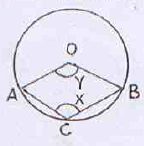Options:

A. 90 degree

B. 2y

C. (180 – y) degree

D. 120 degree

JSTSE Chapter wise Questions of Mathematics Ques No 5:

If D, E, F are mid points of sides BC, CA and AB respectively of a triangle ABC, then the ratio of ar(ABDE) and ar(ABC) is

Options:

A. 1 : 2

B. 2 : 3

C. 3 : 4

D. none of above

## JSTSE Question Papers : Mathematics Chapter 14 : Statistics

Some of questions of JSTSE Maths Question Paper Chapter 14: Statistics as below

JSTSE Chapter wise Questions of Mathematics Ques No 1:

The mean of 13 observation is 14. If the mean of first 7 observation is 12 and the mean of last 7 observation is 16, then the 7th observation is

Options:

A. 12

B. 13

C. 14

D. 16

JSTSE Maths Question Paper Ques No 2:

The value of x so that the mode of the data is 7.

3, 5, 6, 7, 5, 4, 7, 5, 6, x, 8, 7,

Options:

A. 5

B. 6

C. 7

D. 8

JSTSE Chapter wise Questions of Mathematics Ques No 3:

The following data has been arranged in ascending order 24, 27, 28, 31, 34, x, 37, 40, 42, 45. If the median of the data is 35, then the value of x is

Options:

A. 37

B. 36

C. 35

D. 34

JSTSE Maths Question Paper Ques No 4:

If the mean of 10 observations is 61.4 and median is 63, then the mode will be

Options:

A. 63

B. 64.6

C. 66.2

D. 62.2

JSTSE Chapter wise Questions of Mathematics Ques No 5:

The sum of deviations of a set of n values of x1, x2, x3, …, xn measured from 15 and -3 are -90 and 54 respectively. Then the value of n is

Options:

A. 12

B. 8

C. 17

D. 6

For all questions of JSTSE Maths Question Paper Chapter 14: StatisticsClick Here

## JSTSE Question Papers : Mathematics Chapter 15 : Surface Area & Volume

Some of questions of JSTSE Maths Question Paper Chapter 15: Surface Area & Volume as below

JSTSE Chapter wise Questions of Mathematics Ques No 1:

The area of a circular ring enclosed between two concentric circles is 286 cm2. If the difference between their radii is 7 cm, then the radii of these circles are

Options:

A. 12 cm and 5 cm

B. 9 cm and 2 cm

C. 11 cm and 4 cm

D. 10 cm and 3 cm

JSTSE Maths Question Paper Ques No 2:

The surface area of a sphere and a cube are equal. Then the volumes are in the ratio is

Options:

A. 6 : √π

B. √6 : √π

C. 6 : π

D. √6 : π

JSTSE Chapter wise Questions of Mathematics Ques No 3:

If volume of a cube is V units3, the surface area is A units2 and the length of a diagonal is D units. Then

Options:

C. 3A = VD

D. 6D = AV

JSTSE Maths Question Paper Ques No 4:

A hollow sphere with internal and external radius as 7 cm and 8 cm respectively is melted and recast into a cone with a height of 16 cm. The diameter of the base of the cone will be

Options:

A. 26 cm

B. 6.5 cm

C. 13 cm

D. 10 cm

JSTSE Chapter wise Questions of Mathematics Ques No 5:

A conical tent is 12 m high and the radius of its base is 9 m. What is the cost of canvas required to make the tent, if the cost of 1m2 canvas Rs. 14?

Options:

A. Rs. 5840

B. Rs. 5940

C. Rs. 4950

D. Rs. 5960

For all questions of JSTSE Maths Question Paper Chapter 15: Surface Area & VolumeClick Here

## JSTSE Question Papers : Mathematics Chapter 16 : Triangles

Some of questions of JSTSE Maths Question Paper Chapter 16: Triangles as below

JSTSE Chapter wise Questions of Mathematics Ques No 1:

It is given that ∆ABC ≅ ∆FDE and AB = 5 cm, ∠B = 40 degree and ∠A = 80 degree, then which of the following is true?

Options:

A. DF = 5 cm, ∠F = 60 degree

B. DF = 5 cm, ∠E = 60 degree

C. DE = 5 cm, ∠E = 60 degree

D. DE = 5 cm, ∠D = 40 degree

JSTSE Maths Question Paper Ques No 2:

In the figure, X and Y are midpoints of AB and AC respectively and BC = 6 cm, AB = 5.4 cm, AC = 5 cm. Then the perimeter of the trapezium XYCB isOptions:

A. 16.4 cm

B. 14 cm

C. 14.2 cm

D. 13.4 cm

JSTSE Chapter wise Questions of Mathematics Ques No 3:

In the adjoining figure, ABCD is a rectangle and E and F are the midpoints of the side BC and CD respectively. What is the ratio of the area of ∆AEF to the area of ∆FCE?Options:

A. 2 : 1

B. 5 : 2

C. 3 : 1

D. 2 : 3

JSTSE Maths Question Paper Ques No 4:

In the figure, CD and BD are bisectors ∠C = ∠ABE respectively. If ∠BDC = 28 degree, then the value of (∠ABC + ∠ACB) is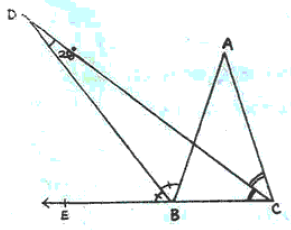Options:

A. 28 degree

B. 56 degree

C. 84 degree

D. 124 degree

JSTSE Chapter wise Questions of Mathematics Ques No 5:

The difference between the sides at right angle in a right angled triangle is 14 cm. The area of the triangle is 120 cm2. The perimeter of the triangle is

Options:

A. 50

B. 36

C. 60

D. 34

For all questions of JSTSE Maths Question Paper Chapter 16: TrianglesClick Here

JSTSE
Previous Year Question Papers
2007-082008-092009-102010-112011-12
2012-132013-142014-152015-162016-17
2018-19PhysicsChemistryMathsBiology
error: Content is protected !!